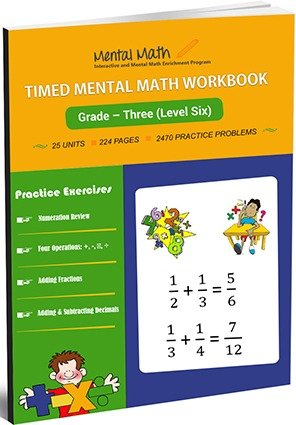15,655## Timed Mental Math Worksheets - Level Six

Students are introduced to the concepts of multiplication and division in this level. Students learn to multiply 3 or more digits by 1 digit number in this level. Students are introduced to the concept of division relating with the concept of multiplication. Division without or with remainders in horizontal and vertical form to dividing 3 digits by 1 digit number are included in this level.

Leave a Review

Worksheet # Topics/Curriculum # of Pages Sample
Unit-1 Learning Numeration 10 pagesUnit-2 Problems on Addition and Subtraction of three Numbers 10 pagesUnit-3 Addition or Subtraction of 2 Digit Numbers 10 pagesUnit-4 Subtracting Tens from Hundred 10 pagesUnit-5 Subtracting 3 Digit Numbers from 3 Digit Numbers 10 pagesUnit-6 Multiplication by 7 to 9 10 pagesUnit-7 Multiplying 2 Digit Numbers by 2 through 9 10 pagesUnit-8 Multiplying 2 Digit Numbers by 1 Digit Numbers 10 pagesUnit-9 Multiplying 3 or More Digit Numbers by 1 Digit Numbers 10 pagesUnit-10 Dividing the Numbers up to 50 by 2 through 9 10 pagesUnit-11 Dividing the Numbers up to 90 by 2 through 9 10 pagesUnit-12 Dividing the Numbers up to 99 by 2 through 9 in vertical form 10 pagesUnit-13 Dividing 3 Digit Numbers by 1 Digit Numbers up to 500 10 pagesUnit-14 Dividing 3 Digit Numbers by 1 Digit Numbers up to 999 10 pagesUnit-15 Multiplying 2 Digit Numbers by 2 Digit Numbers 10 pagesUnit-16 Numeration Review 10 pagesUnit-17 Number Concept 10 pagesUnit-18 Dividing 2 or 3 Digit Numbers by 2 Digit Numbers 10 pagesUnit-19 Understanding Fractions 10 pagesUnit-20 Introducing Fractions 10 pagesUnit-21 Adding Fractions with Like Denominator 10 pagesUnit-22 Addition of Decimals 10 pagesUnit-23 Subtraction of Decimals 10 pagesUnit-24 Geometry 10 pagesUnit-25 Measurement: Area, Perimeter, and Volume 10 pages